Next: 5.3.2 Fitting Procedure Up: 5.3 Determination of the Parameter Set for the Simulation Previous: 5.3 Determination of the Parameter Set for the Simulation

5.3.1 Identification of the Most Critical Parameters

A figure of merit for HEMTs is the maximum transconductance gm max. One of the most important parameters governing gm max is the gate-to-channel separation dGC. The thickness of the epitaxial layers can be controlled very precisely by MBE growth. The critical technological step which determines the magnitude of dGC is the gate recess. In the recess region it is intended to remove the GaAs cap layer completely but to leave the AlGaAs Schottky barrier intact. In practice, this etching can only be performed with a finite selectivity, and the effective recess depth can vary a few nanometers across the wafer or from on processing run to another. Therefore, small deviations in the order of 2 nm of the actual dGC from the nominal values must be considered to be realistic. Deviations of the gate length LG from their nominal values are also technological inevitable. These deviations highly depend on the used technology to define the gate structure.

First the impact of the most important parameters on the intrinsic device should be investigated using an analytical formula. The intrinsic gm max int (i. e. gm max for vanishing source resistance RS) of a HEMT is given by :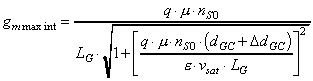. (60)

Here, e, ns0, m, and vsat are the permittivity of the semiconductor, the equilibrium electron sheet concentration, the low field mobility, and the saturation velocity in the channel respectively, and DdGC is the distance between the channel/barrier heterointerface and the maximum of the electron probability distribution in the channel.

 µ [m2/Vs] vsat [m/s] LG [nm] dgc [nm] ns0 [cm-2] 0.6 1.5*105 250 20 1*1012

The parameters m and vsat depend on the channel material whereas LG and dGC are geometry quantities defined by process technology. The equilibrium electron concentration ns0 depends on various parameters such as doping concentration in the barriers, the distance of the doping layer to the channel, and the height of the energy barriers. For practical devices with typical values ns0 this will have almost no influence on gm max int as shown in  Figure 5.15 but only determine the threshold voltage VT. In the following gm max int will be plotted versus the parameters m, vsat, LG, and dGC to qualitatively analyze what has to be expected for the measured and simulated devices investigated in Chapter 6. Typical values of an Al0.2Ga0.8As/In0.2Ga0.8As HEMT used for the plots are given in Table 5.1. The parameters which are not varied actually are kept at these constant values.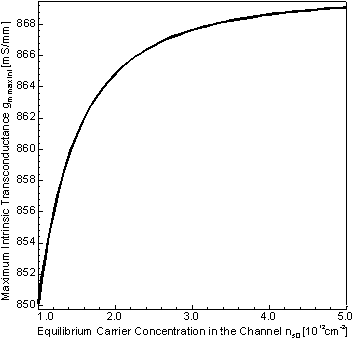Figure 5.15 gm max int versus the equilibrium sheet carrier concentration ns0.

The magnitude of the material parameter m can be determined by Hall measurements. The absolute measured values have fairly large uncertainties because there is no standardized epitaxial Hall structure.  Figure 5.16 reveals that gm max int is nearly independent of m for LG < 250 nm and m higher than about 4000 cm2/Vs. With increasing LG the influence of m increases.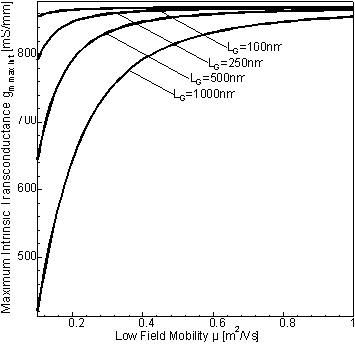Figure 5.16 gm max int versus the electron low field mobility for different gate lengths.

The experimental determination of vsat is even more difficult than that of µ and can be done directly only for bulk material. When the same material is used in a quantum well additional effects have to be taken into account. These are the change in alloy scattering depending on the growth technique, interface roughness, Coulomb scattering, electron scattering and change in the v(E) characteristics due to quantization effects. Today there are no models suitable for device simulation which can describe these effects in AlGaAs/InGaAs quantum wells. It has to be expected that vsat is reduced significantly compared to the bulk material values. Therefore especially vsat is considered to be a fitting parameter. In  Figure 5.17 the dependency on vsat is shown. Practical devices operate in the saturation region when they reach their maximum gm. Therefore gm max int is almost linearly dependent on vsat as expected.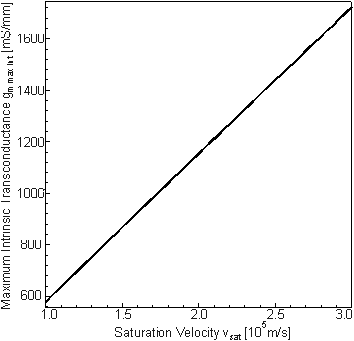Figure 5.17 gm max int versus the saturation velocity of the electrons in the channel.

The dependence of gm max int on LG is plotted in  Figure 5.18. For LG < 250 nm only a small influence can be observed. The decrease is almost linear for LG > 500 nm. The expected reduction proportional to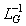occurs for gate lengths of several microns (not shown in  Figure 5.18).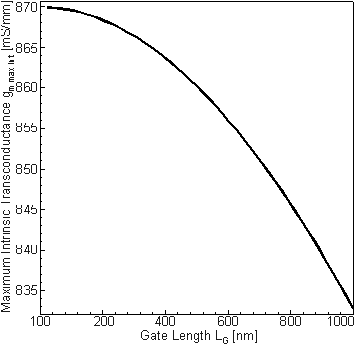Figure 5.18 gm max int versus the gate length.

As shown in  Figure 5.19 the dependence of gm max int on (dGC + DdGC) is only moderate for values larger than about 30 nm. It appears that gm max int gets extremely sensitive to (dGC + DdGC) for much smaller values which nowadays can be manufactured with an accuracy below 2 nm. Considering (dGC + DdGC) = 20 nm a reduction of 1 nm (which corresponds to only 4 atomic layers) increases gm max int by about 50 mS/mm. This establishes dGC as the most important technological parameter for the transconductance of HEMTs with LG below 1 µm which are nowadays used for practical applications.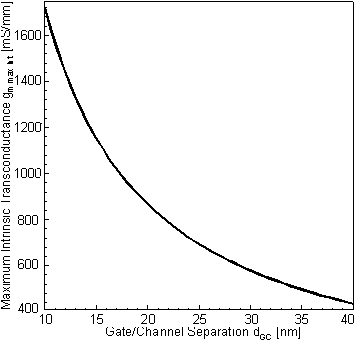Figure 5.19 gm max int versus the gate to channel separation.

Helmut Brech
1998-03-11